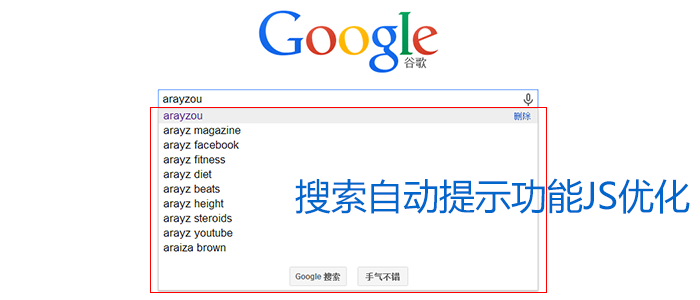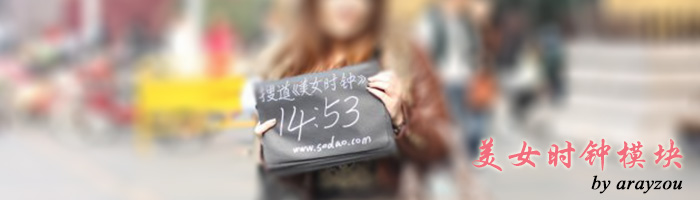0%``````
\$('textarea').autosize();
});
```
```

jQuery判断函数是否存在代码：

``````
//判断delectTag()函数是否存在
if (\$.isFunction(window.delectTag)){
delectTag();
}
```
```

``````// 获得cookie

// 删除

``````var date = new Date();
var minutes = 10;
date.setTime(date.getTime() + (minutes * 60 * 1000));``document.onkeydown=function(e){    var isie = (document.all) ? true:false;    var key;    if(isie){        key = window.event.keyCode;    }else{        key = e.which;    }    if(key==37){        alert(‘left’);    }    if(key==38){        alert(‘top’);    }    if(key==39){        alert(‘right’);    }    if(key==40){        alert(‘down’);    }};``